Want to share your content on R-bloggers? click here if you have a blog, or here if you don't.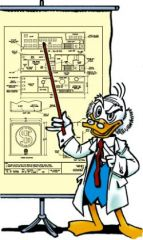A standard idea in extreme value theory (see e.g. here, in French unfortunately) is that to estimate the 99.5% quantile (say), we just need to estimate a quantile of level 95% for observations exceeding the 90% quantile.

In extreme value theory, we assume that the 90% quantile (of the initial distribution) can be obtained easily, e.g. the empirical quantile, and then, for the exceeding observations, we fit a Pareto distribution (a Generalized Pareto one to be precise), and get a parametric quantile for the 95% quantile. I.e.which can be written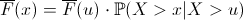So, an estimation of the cumulative distribution function isand if we invert it, we get the popular expression for high level quantiles,Hence, we do not really care about observations in the core of the distribution.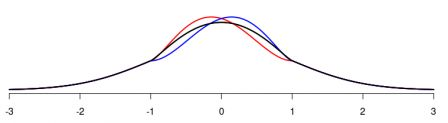And I was wondering if this can be transposed with quantile regressions. Hence, I would like to get a quantile regression of level 90% (say) ofgiven, based on observations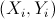‘s, but all observations such that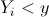for someare missing. More precisely, I have the following sample (here half of the observations are missing),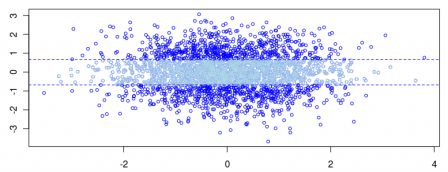Assume that we know that I have observations below thequantile of level 25%, and above thequantile of level 75%.
If I want to get the 90% quantile regression, and the 10% quantile, the code is simply,
```library(mnormt)
library(quantreg)
library(splines)
set.seed(1)
mu=c(0,0)
r=0
Sigma <- matrix(c(1,r,r,1), 2, 2)
Z=rmnorm(2500,mu,Sigma)
X=Z[,1]
Y=Z[,2]

base=data.frame(X,Y)
plot(X,Y,col="blue",cex=.7)
I=(Y>qnorm(.25))&(Y<qnorm(.75))
baseI=base[I==FALSE,]
points(X[I],Y[I],col="light blue",cex=.7)
abline(h=qnorm(.25),lty=2,col="blue")
abline(h=qnorm(.75),lty=2,col="blue")
u=seq(-5,5,by=.02)
reg=rq(Y~X,data=base,tau=.05)
lines(u,predict(reg,newdata=data.frame(X=u)),lty=2)
reg=rq(Y~X,data=baseI,tau=.05*2)
lines(u,predict(reg,newdata=data.frame(X=u)))```
The graph is the followingDotted lines - in black - are theoretical lines (if I had all observations), and plain lines are (where half of the sample if missing). Instead of a standard linear quantile regression, it is also possible to try a spline regression,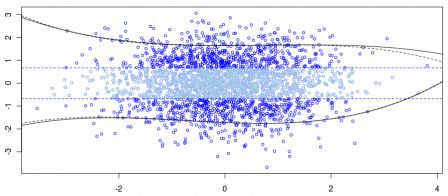So obviously, if I miss something in the middle, that's no big deal, doted and plain lines are here extremely close.
But what if observationsandwere correlated ? Consider a Gaussian random vectorwith correlation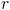(here 0.6).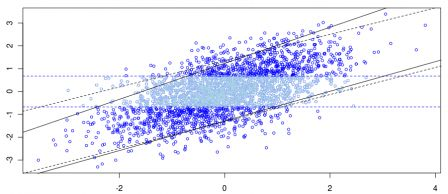It looks like we overestimate the slope for high quantile, but not for lower quantiles. So if observations are correlated, we have to be cautious with that technique.
But why could that be interesting ? Well, because I wanted to run a quantile regression on marathon results. But I could not get the overall dataset (since I had to import observations manually, and I have to admit that it was a bit boring). So I extracted finish times of the first 10% athletes, and the latest 10%. And I was wondering if it was enough to look at the 5% and 95% quantiles, based on the age of the runner... To be continued.